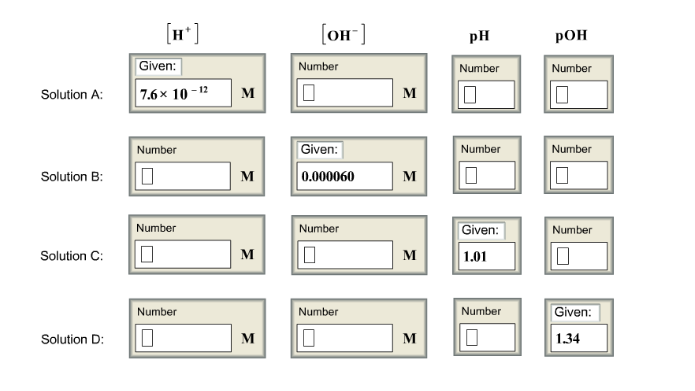# Problem: Complete this table of values for four aqueous solutions at 25°c

###### FREE Expert Solution
99% (211 ratings)
###### FREE Expert Solution

pH  of a solution is found by: pH = -log[H+]

pOH of a solution is found by: pOH = -log[OH-]

The relationship between pH and pOH is: pH + pOH = 14

[H+] [OH-] = 1.0 x 10-14

For solution A:

The concentration of [H+ given is 7.6x10-12M

To find pH:

pH = -log[H+]          pH = -log [7.6x10-12]          pH = 11.12

99% (211 ratings)###### Problem Details

Complete this table of values for four aqueous solutions at 25°cWhat scientific concept do you need to know in order to solve this problem?

Our tutors have indicated that to solve this problem you will need to apply the pH and pOH concept. You can view video lessons to learn pH and pOH. Or if you need more pH and pOH practice, you can also practice pH and pOH practice problems.

What is the difficulty of this problem?

Our tutors rated the difficulty ofComplete this table of values for four aqueous solutions at ...as medium difficulty.

How long does this problem take to solve?

Our expert Chemistry tutor, Sabrina took 10 minutes and 52 seconds to solve this problem. You can follow their steps in the video explanation above.

What professor is this problem relevant for?

Based on our data, we think this problem is relevant for Professor Islam's class at UIC.